0
1137

# Directions Question for RRB Group-D PDF

Download Top-15 RRB JE Directions Questions PDF. RRB GROUP-D General Reasoning questions based on asked questions in previous exam papers very important for the Railway Group-D exam.

Question 1: Manish started from his home and headed east. He walked for 4 km and then took a right turn. He walked for another 3 km and then took a left turn. After travelling for another 4 km, he takes another left and walks for 3 km. What is the shortest distance of his current position from his home?

a) $7\sqrt{2}$ km

b) 8 km

c) $5\sqrt{3}$ km

d) 10 km

Question 2: A person first travels towards North $3\frac{1}{2}$ km, then he turns left and travel $1\frac{1}{2}$ km and then turns towards left and travel for $3\frac{1}{2}$ km. How far is he from the starting point ?

a) 2km West

b) $1\frac{1}{2}$ km West

c) $2\frac{1}{2}$ km East

d) None

Instructions

If you start running from a point towards North and after covering 4 km you turn to your left and run 5 km and then turn to your left and run 5 km and then turn to left and again and run another 6 km and before finishing you take another left turn and run 1 km, then answer questions based on this information.

Question 3: From the finishing point if you have to reach the point from where you started in which direction will you have to run ?

a) East

b) West

c) North

d) South

Question 4: After taking the second turn, in which direction will you be running ?

a) East

b) West

c) North

d) South

Question 5: In which direction will you be running while finishing ?

a) East

b) West

c) North

d) South

Question 6: How many km are you from the place you started ?

a) 1 km

b) 2 km

c) 3 km

d) 4 km

Question 7: If the north west direction becomes south, then which direction’s place will south take?

a) North

b) South-East

c) East

d) North-East

Question 8: Ramesh walks a certain distance from his home. He takes a right turn and walks certain distance. He then takes a left turn and finally a left turn once more to face north. Which direction was he facing initially?

a) North

b) South

c) East

d) West

Question 9: A bus moves 3km in east direction from its starting point . It then turns south and moves 4km . It again turns east and move 5km. It then turns north and moves 4km. It finally moves 6km to the east to reach ending point. Find the distance between the starting point and the ending point.

a) 12km

b) 22km

c) 14km

d) 18km

Question 10: A bus starts in a certain direction and takes a left turn. It then takes a right turn after travelling some distance and finally takes one more right turn. Which direction is the bus facing if it started facing East?

a) South

b) East

c) North

d) West

Question 11: Rohit walks 3km north from his home. He then takes a left turn and walks some distance. Then he turns right. Which direction is he facing now?

a) North

b) South

c) East

d) West

Question 12: Jeff start from his home and walks 8km to the south. He then takes a left turn and walks 6km. He finally turns left and walks 16km to reach his office. Find the distance between his home and office?

a) 10km

b) 8km

c) 12km

d) 14km

Question 13: A bus starts from point P and moves north. It then takes a left turn and moves some distance. It again takes a left turn and moves certain distance. It finally takes a right turn to reach its destination. Find the direction in which the bus is facing now?

a) North

b) South

c) East

d) West

Question 14: Arunesh walks 5km north. He then takes right and walks 5km. He again takes right and walks 17km to reach his destination. Find the distance between starting point and ending point?

a) 13km

b) 22km

c) 20km

d) 10km

Question 15: Ramesh starts from point O towards north and walks 12km. He then turns east and moves 5km to reach B. Find the distance between O and B?

a) 17km

b) 13km

c) 14km

d) 10km

The given situation can be reflected through the following diagram.

From this, we can see that that the shortest distance between the given points will be
d = 4 + 4 = 8 kmDistance from starting point= VS=1.5km West

The information given is depicted belowTo travel from U to S, we have to travel West.

The information given is depicted belowIf we take the 2nd turn, we will be running from U to V i.e in the South Direction.

The information given is depicted belowWhile finishing, we will be running from T to U, which is the North direction.

The information given is depicted belowThe distance between the starting point and ending point is SU which is 1 km.

Refer to the following diagram.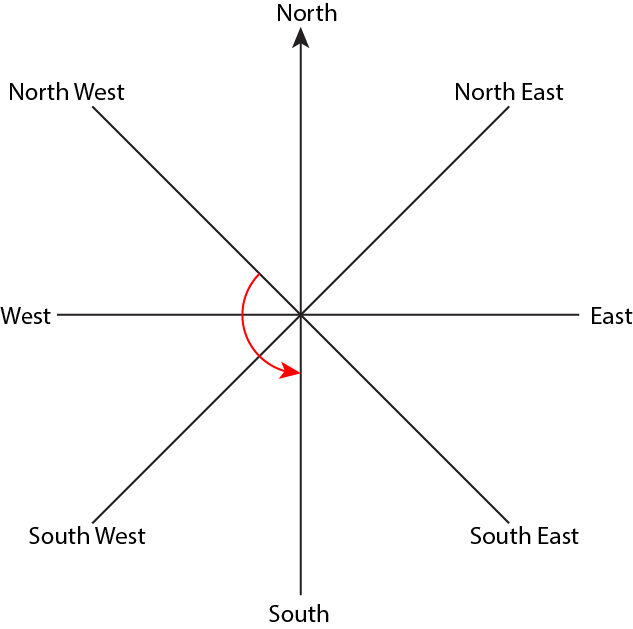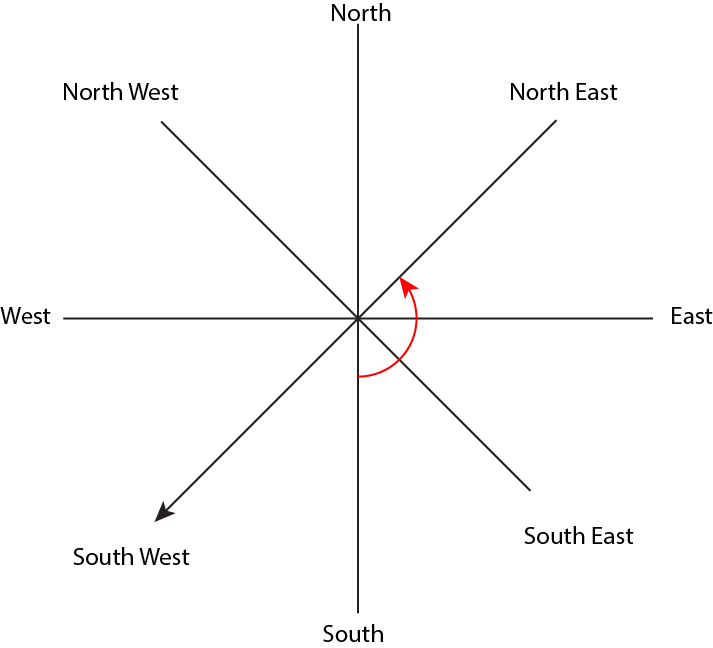When north-west direction becomes south, the axes rotate by 135 degrees in a counter-clockwise direction. All the directions will rotate in this way only. North East is 135 degrees counter clockwise to south.

In this question, we have to move backwards starting from the final position of facing North. He took 1 right and 2 left from his initial position. Thus, we have to take 2 rights and 1 left to reach his initial position.

East is to the right of North and South is to the right of East. East is to the left of South. Hence, the direction Ramesh was initially facing was East. The diagram is as shown below. Here O is starting point and A is ending point.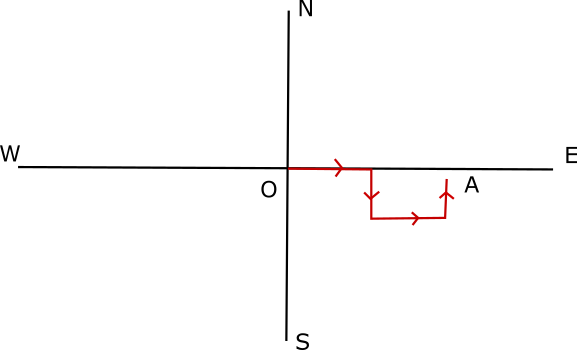The final distance between the starting point and the ending point is (3+5+6)km = 14km as shown.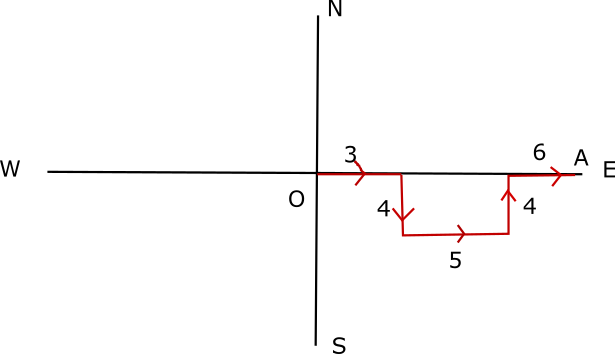Here O is the starting point and A is the ending point.

The bus starts facing East and takes a left turn. North is to the left of East. After travelling towards North for some distance, it takes a right turn. East is to the right of North. Finally, it takes one more right turn while facing East. South is to the right of East. Hence, the bus is facing South.

The diagram is as shown. O is starting point and A is ending point.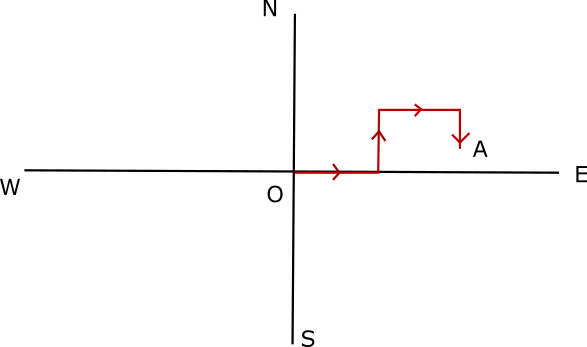Rohit walks towards North and then takes a left. West is to the left of North. After walking towards West, he takes a right turn. North is to the right of West. Hence, he is facing North.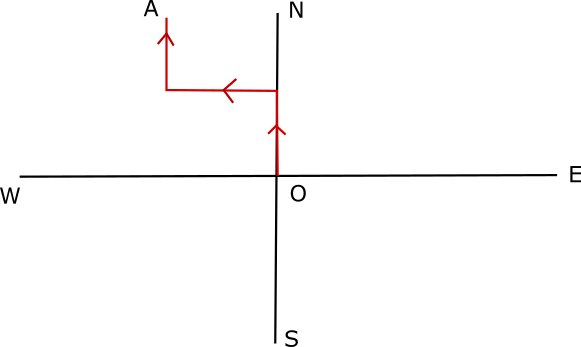Jeff firstly walks 8km to the south. He then takes a left turn to face east and walks 6km. He then turns left to face north and walks 16km as shown. The diagram is as shown. O is the starting point and A is the ending point. We have to calculate OA.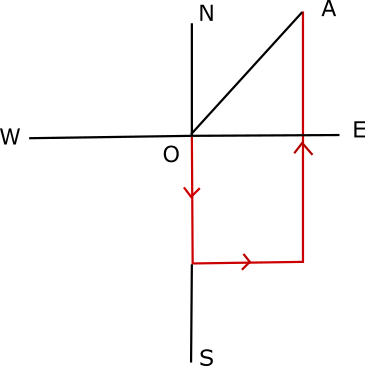Distance between home and office =$\sqrt{(16-8)^2+6^2}$ = 10km

Firstly the bus moves north. It then takes a left turn. As discussed in the concept, West is to the left of North. The bus moves west.
Now the bus again takes a left turn. South is to the left of West. So the bus is facing South.
It finally takes a right turn. West is to the right of South. So the bus would be facing west. The diagram is as shown below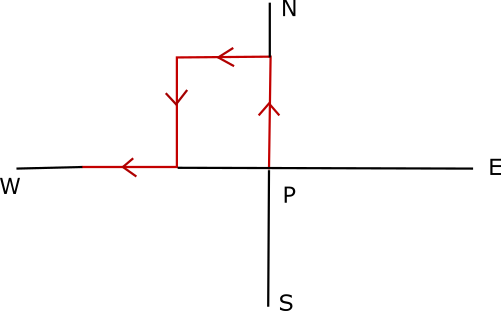Arunesh walks 5km north. He then takes right turn to walk 5m east. He then again takes right turn to walk 17 km south to reach his destination. The diagram is as shown below. The arrowheads show the path of Arunesh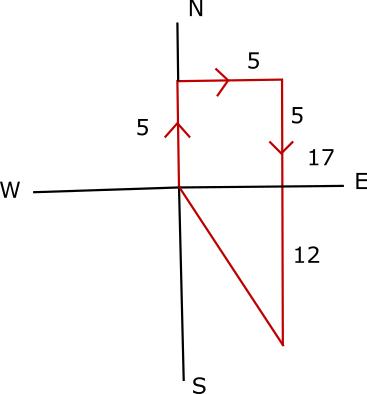Distance =$\sqrt{(17-5)^2+5^2}$ = 13km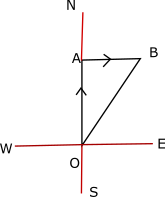Net distance =$\sqrt{12^2+5^2} = 13km$